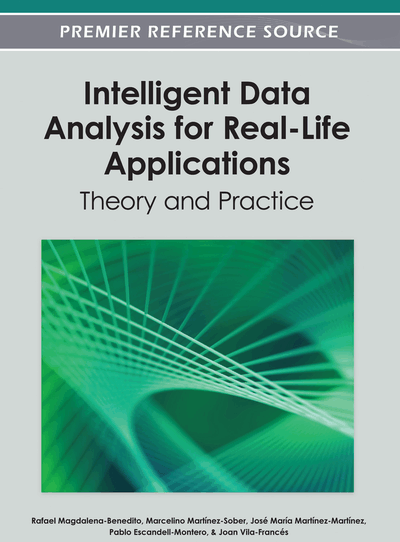# Gaussian Process-based Manifold Learning for Human Motion Modeling

Guoliang Fan (Oklahoma State University, USA) and Xin Zhang (South China University of Technology, P. R. China)
DOI: 10.4018/978-1-4666-1806-0.ch015
Available
\$37.50
No Current Special Offers

## Abstract

This chapter studies the human walking motion that is unique for every individual and could be used for many medical and biometric applications. The authors’ goal is to develop a general low-dimensional (LD) model from of a set of high-dimensional (HD) motion capture (MoCap) data acquired from different individuals, where there are two main factors involved, i.e., pose (a specific posture in a walking cycle) and gait (a specific walking style). Many Gaussian process (GP)-based manifold learning methods have been proposed to explore a compact LD manifold embedding for motion representation where only one factor (i.e., pose) is normally revealed explicitly with the other factor (i.e., gait) implicitly or independently treated. The authors recently proposed a new GP-based joint gait-pose manifold (JGPM) that unifies these two variables into one manifold structure to capture the coupling effect between them. As the result, JGPM is able to capture the motion variability both across different poses and among multiple gaits (i.e., individuals) simultaneously. In order to show advantages of joint modeling of combining the two factors in one manifold, they develop a validation technique to compare JGPM with recent GP-based methods in terms of their capability of motion interpolation, extrapolation, denoising, and recognition. The experimental results further demonstrate advantages of the proposed JGPM for human motion modeling.
Chapter Preview
Top

## Introduction

Human motion analysis is becoming a popular research topic due to its wide applications such as human detection, tracking, recognition, and 3D character animation etc. It is also a challenging research topic due to the high-dimensional (HD), non-linear, and multi-factor nature of human motion data from different individuals or activities. In this work, we focus on the walking motion, i.e., the gait. Many Gaussian process (GP)-based manifold learning algorithms have been proposed to explore the low-dimensional (LD) latent structures from the HD visual or kinematic data, by which the problems of motion analysis or pose estimation can be well constrained. Specifically, the pose manifold is often used to represent the cyclic nature of a gait and can be learned from either kinematic data (Urtasun, Fleet, & Fua, 2006), (Gupta, Chen, Chen, Kimber, & Daivs, 2008), (Ek, Torr, & Lawrence, 2007) or visual data (Elgammal & Lee, 2004a), and the view manifold was also involved in (Lee & Elgammal, 2007) by which it is possible to interpolate gait observations for new views during pose estimation. Moreover, when multiple motion styles from different subjects or activities are involved, two independent style-related linear variables, “identity” and “gait’, (Wang, Fleet, & Hertzmann, 2007) or a discrete “style” variable (Elgammal & Lee, 2004b) or separate motion trajectories (Gupta, et al., 2008) were used.

In our early work (Zhang & Fan, 2010), the idea of gait manifold was proposed to capture the motion variability among different individuals, by which we also define a continuous-valued gait variable that can be used to extrapolate new gait motions via nonlinear interpolation along the gait manifold. Specifically, the gait and pose manifolds were treated separately for motion modeling where the pose and gait variables are assumed to be independent. Instead, our studies revealed that the two variables may be coupled and should be jointly considered to specify a particular gait motion sequence. Therefore, we suggested a joint gait-pose manifold (JGPM) recently (Zhang & Fan, 2011) that is learned via a Gaussian process (GP)-based manifold learning method that has a probabilistic representation for robust motion analysis, unlike the one in (Zhang & Fan, 2010) where both the pose and gait manifolds are learnt as the deterministic structure.

The proposed JGPM was intended not only to reflect the coupling relationship between the pose and gait variables, but also to preserve their own manifold structures. It was assumed in our early work (Zhang & Fan, 2010) that the gait manifold has a closed-loop 1D structure while the pose manifold is characterized by a circle. Inspired by (Elgammal & Lee, 2009) where a torus is used to represent multi-view dynamic gait observations featured with two cyclic variables (i.e., pose and view), we suggested a toroidal structure for JGPM (Zhang & Fan, 2011), as shown in Figure 1. Moreover, three versions of JGPM were developed to examine the validity of the assumption that JGPM may follow a toroidal structure, i.e., torus-based (JGPM-I), torus-constrained (JGPM-II), and torus-like (JGPM-III). The first involves a directly nonlinear radial basis function (RBF)-based mapping like the one in (Elgammal & Lee, 2009) without probabilistic learning that serves as a baseline reference. The second is learned via a method extended from a recent topologically-constrained GP algorithm but still remains an ideal torus that is an idealized JGPM with an optimized latent space. The third is learned via a new two-step GP algorithm that tends to balance the ideal structure assumption with the actual intrinsic data structure. It was shown that torus-like JGPM not only outperforms the recent GP algorithms (N. Lawrence & J. Candela, 2006), (K. Grochow, S. Martin, A. Hertzmann, & Z. Popovic, 2004), (Urtasun, et al., 2006), (Wang, et al., 2008) in terms of synthesizing new gait motions (i.e., extrapolation), but also improve video-based motion estimation compared with (Zhang & Fan, 2010). The major advantage of JGPM-III is its compact, well-organized and smooth latent space that is nicely supported by the torus-like manifold surface, unlike its peers where the latent space is normally relative sparse and less-organized.

## Complete Chapter List

Search this Book:
Reset